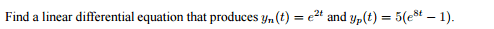# Finding a linear differential equation

## Homework Statement## The Attempt at a Solution

So the general solution is the sum of the null solution (Yn) and particular solution (Yp)

I believe I just need to write:

y = e2t + 5e8t - 5 + C

and then find the derivative of both sides

y' = 2e2t + 40e8t

is this correct?

Mark44
Mentor

## Homework Statement

View attachment 101985

## The Attempt at a Solution

So the general solution is the sum of the null solution (Yn) and particular solution (Yp)

I believe I just need to write:

y = e2t + 5e8t - 5 + C
This is almost the general solution. It would be y(t) = Ae2t + 5e8t - 5.
The first part is the complementary solution (the solution to the homogeneous diff. equation) -- what you're calling the null solution. The second part is the particular solution, a solution to the nonhomogeneous problem.
goonking said:
and then find the derivative of both sides

y' = 2e2t + 40e8t

is this correct?
No, and it's nowhere near close.
What you're supposed to do is find the nonhomogeneous differential equation that has y(t) as its solution.Your textbook should have some examples that are similar to this problem. That should be your first resource.

•goonking